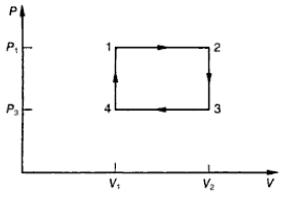Q.1
• 2 μ F
• 4μ F
• 3μ F
• 1 μ F
Q.2
##### A certain mass of gas is held at pressure P1=2X105 N/m2  and occupy a volume 1 mThe gas expands at constant pressure such that volume becomes doubled( i.e. P2=P1 ,V2=2V1 ). It is then held at constant volume while its pressure is halved ( P3=P2/2 ,V3=V2) . A cyclic transformation is completed by the constant pressure compression ,followed by isobaric transformation as shown in below figure Δ W = Net work done by the gas in the cyclic transformation ΔQ=Net Heat absorbed by the gas• Δ Q=2 Joule, ΔW=2Joule
• ΔQ=4 Joule, ΔW=2Joule
• ΔQ=1 Joule, ΔW=2Joule
• None of these
Q.3
##### A Small solid sphere of radius r can roll without slipping on the inner surface of a fixed large spherical shell of Radius R . Find the period of small oscillation of small sphere around its equilibrium position?
• $2 \pi \sqrt {((2(R-r))/5g)}$
• $2\pi \sqrt {((7(R+r))/5g)}$
• $2\pi \sqrt {((7(R-r))/5g)}$
• 2\pi \sqrt {((7(R-r))/2g)}
Q.4
• A2 : B2
• A2 : C2
• C:A
• 1:1
Q.5
##### Three bodies form an isolated system. There are m1 =m, m2 = 2m and m3 = 3m. They have different direction, but all have the same initial speed vOne or more elastic collision between the pair of the bodies where otherwise do not interact. Find the maximum possible final speed of each of the three bodies.
• 3 v0, 2 v0, v0
• v0, 2 v0, 3 v0
• 2.4 v0, 1.73 v0, 1.41 v0
• None of the above
Q.6
##### A conductor of non uniform curvature is given Q charge. Which of the following is correct?
• The charge is distributed uniformly over its volume
• The charge is distributed uniformly over its outer surface
• The charge has the greatest concentration on the part of greatest curvature
• The charge has the greatest concentration on the part of least curvature
Q.7
• .125V
• .1 V
• .25V
• .8 V
Q.8
##### A particle executes a SHM of amplitude 1.0 cm along the principle axis of a convex lens of Focal length 12 cm. The mean position of oscillation is at 20 cm from the lens. Find the amplitude of the image of the particle?
• 2.3 cm
• 3.2cm
• 4 .1 cm
• None of these
Q.9
##### A capacitor of capacitance C1 = 1 μF withstands the maximum voltage V1 = 6 kV while another capacitance C2 = 2 μF withstands the maximum voltage V2 = 4 kV. What maximum voltage will the system of these two capacitors withstand if they are connected in series?
• 10KV
• 4KV
• 9KV
• None of these
Q.10
• 2 and 2
• 6 and 8
• 4 and 3
• 8 and 6
Q.11
##### Both the light and sound wave suffer diffraction. It is more difficult to observe diffraction with light waves because
• Speed of light is far greater
• Light wave can travel in vacuum
• Light waves are transverse waves
• The wavelength of light waves is far smaller
Q.12
##### A satellite in force free space sweeps stationary interplanetary dust at a rate given by dM/dt=pv Where M is the mass, v is the velocity of the satellite and p is a constant
• pv2
• $v^2/pM$
• $(pv^2)/M$
• (pv )/M